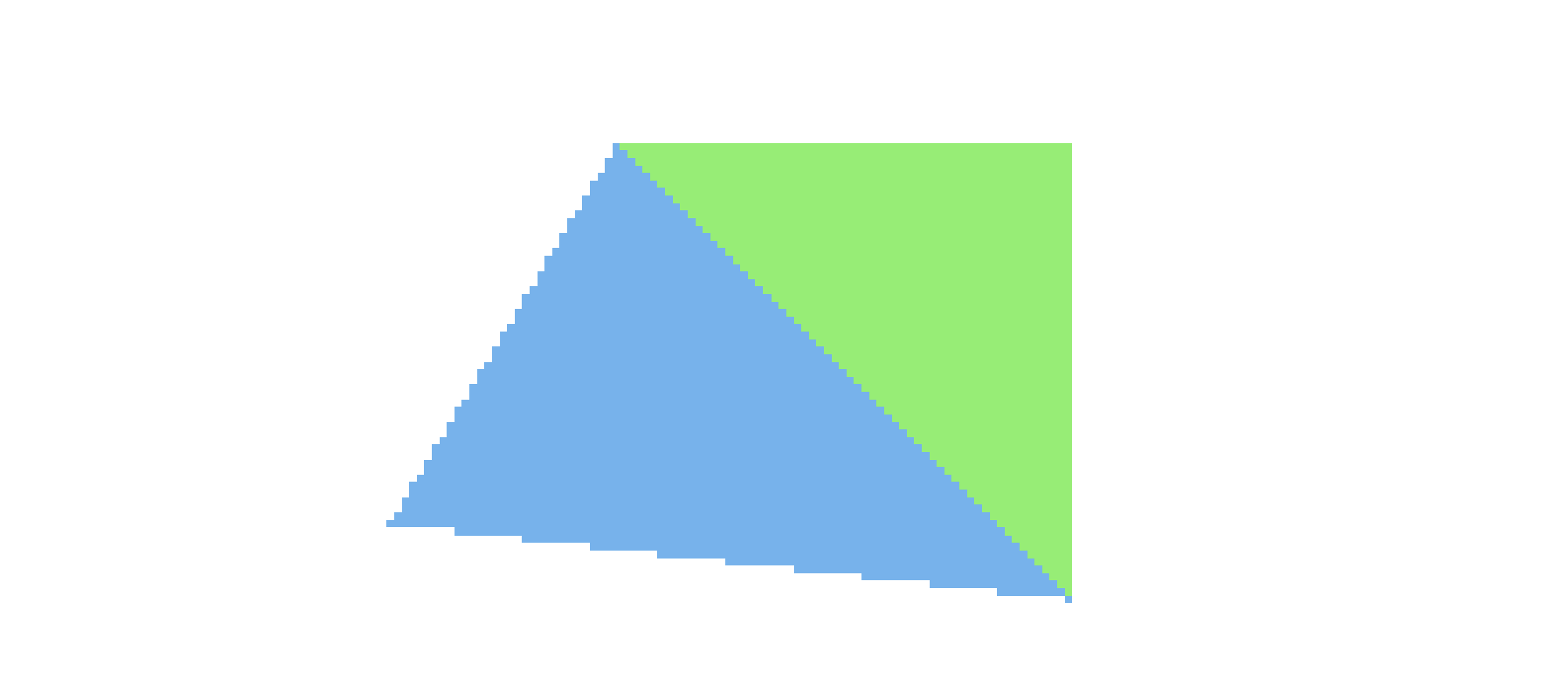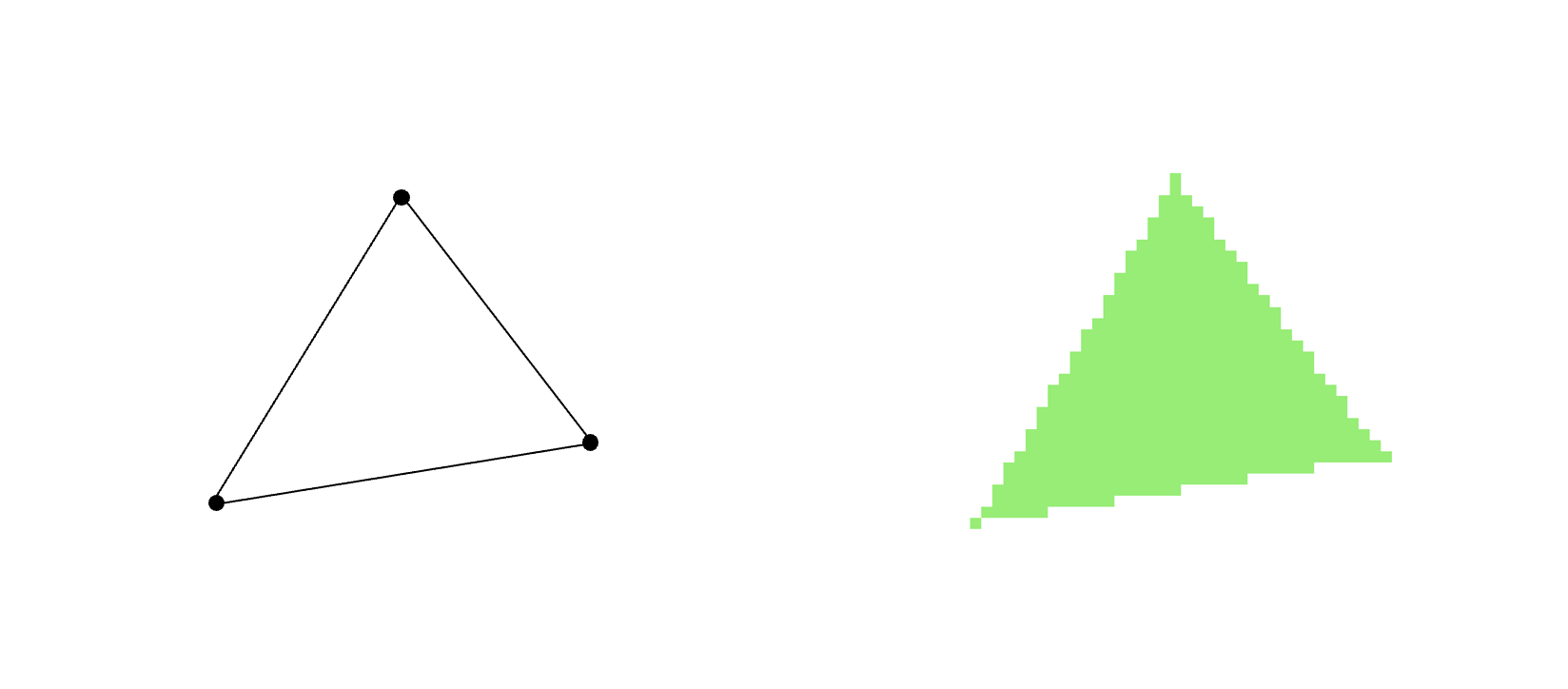Kristoffer Dyrkorn, November 4, 2022

# A fast and precise triangle rasterizerIn this article series, you will get to learn how a computer draws a triangle on the screen. We will create a simplified software implementation of the method GPUs use when they draw (single-colored) triangles, and through example code and small demo apps you will gain insights in the challenges - and solutions - involved in making a fast and precise software rasterizer.

We will use JavaScript as the implementation language, and build everything from scratch. The code will run standalone in any modern browser and should be fairly easy to port to other languages and runtime environments.

The tutorial will work best if you have some knowledge of maths, programming and binary numbers, but otherwise no particular background or skill set is needed.

The main takeaways are:

• an outline of the most common rasterization method
• the problems floating point number representations can introduce
• an intro to the fixed point number representation, and how it relates to subpixels
• incremental calculations in the rasterizer can give a 10x performance gain

But now, let’s get started!

First, let’s have a look at what it means to draw a triangle on the screen - to rasterize a triangle. The word rasterization can be explained this way: A triangle is defined by three points (vertices). If you draw lines between each of them, you get to see the triangle. However, a computer cannot do that - a computer needs to draw pixels. Put differently: To make the computer draw a triangle, we have to tell it to change the colors for a certain set of pixels - that together make up the triangle - on screen. Screen pixels are organized in a regular grid, a raster, and this is what gives us the name: Rasterization is the process of drawing geometry, typically defined by mathematical functions, on a pixel screen. (Our triangles edges can be described by lines - having a mathematical definition such as `y = ax + b`.)The article series is structured as follows: First, you will get to know the principles behind triangle rasterization and the specific approach we will use. We will then make a simple, first version of a rasterizer. It will gradually be refined as we test it out and discover needs for improvement. We will first prioritize correctness - ie that the rasterizer draws exactly the pixels it should. We will then look at performance optimizations. As you will see, the improvements will give us a tenfold performance boost!

## Sections

If you want to test out, modify and run the example code locally, clone this repository, start a local web server in the root directory (for example, using `python3 -m http.server`) and open the web page (at `http://localhost:8000` or similar). Each subfolder has a small demo application you can look at.

If you prefer to just run the example apps, use the links at the end of each section.